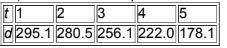×
Get Full Access to Elementary Statistics - 12 Edition - Chapter 10.6 - Problem 5 bsc
Get Full Access to Elementary Statistics - 12 Edition - Chapter 10.6 - Problem 5 bsc

×

# Finding the Best Model. Construct a scatterplot andISBN: 9780321836960 18

## Solution for problem 5 BSC Chapter 10.6

Elementary Statistics | 12th Edition

• Textbook Solutions
• 2901 Step-by-step solutions solved by professors and subject experts
• Get 24/7 help from StudySoup virtual teaching assistantsElementary Statistics | 12th Edition

4 5 1 356 Reviews
30
3
Problem 5 BSC

Statistical Literacy and Critical Thinking

Finding the Best Model. In Exercises 5–16, construct a scatterplot and identify the mathematical model that best fits the given data. Assume that the model is to be used only for the scope of the given data, and consider only linear, quadratic, logarithmic, exponential, and power models.

Dropped Object The table lists the distance d (meters) above the ground for an object dropped in a vacuum from a height of 300 m. The time t (in sec) is the time after the object has been released.Step-by-Step Solution:

Step 1 of 1

In Excel draw the scatter plot for t and d.

Right click on any point, click on ‘add trendline’ option.

Click ‘Linear’ and choose ‘display equation on chart’ and ‘R-squared value’.

LinearLogarithmicExponentialPower modelsConclusion:

Here, the quadratic model that best fits the given data since it has higher R2 value.

Step 2 of 1

##### ISBN: 9780321836960

Elementary Statistics was written by and is associated to the ISBN: 9780321836960. The answer to “?Statistical Literacy and Critical ThinkingFinding the Best Model. In Exercises 5–16, construct a scatterplot and identify the mathematical model that best fits the given data. Assume that the model is to be used only for the scope of the given data, and consider only linear, quadratic, logarithmic, exponential, and power models.Dropped Object The table lists the distance d (meters) above the ground for an object dropped in a vacuum from a height of 300 m. The time t (in sec) is the time after the object has been released.” is broken down into a number of easy to follow steps, and 89 words. This textbook survival guide was created for the textbook: Elementary Statistics, edition: 12. This full solution covers the following key subjects: Object, model, given, Best, dropped. This expansive textbook survival guide covers 121 chapters, and 3629 solutions. Since the solution to 5 BSC from 10.6 chapter was answered, more than 283 students have viewed the full step-by-step answer. The full step-by-step solution to problem: 5 BSC from chapter: 10.6 was answered by , our top Statistics solution expert on 03/15/17, 10:30PM.

Unlock Textbook Solution